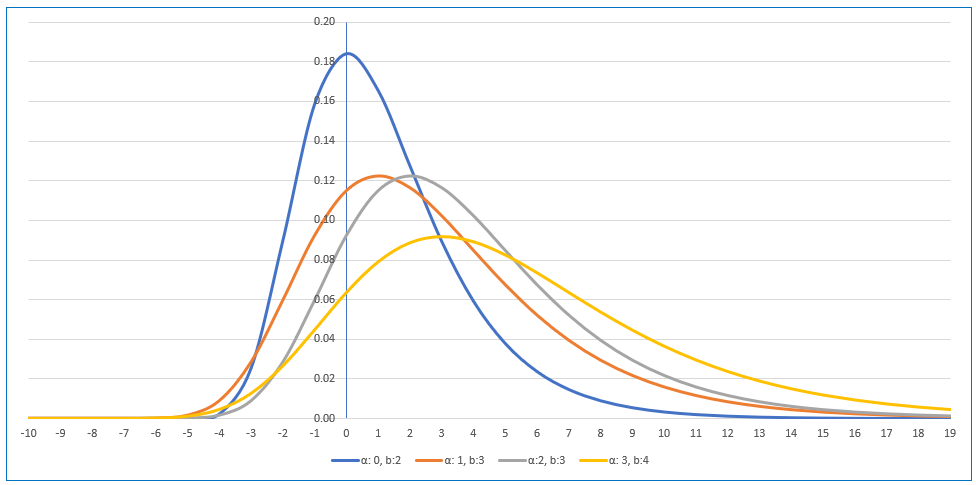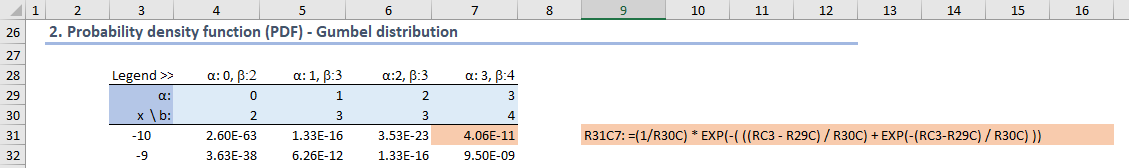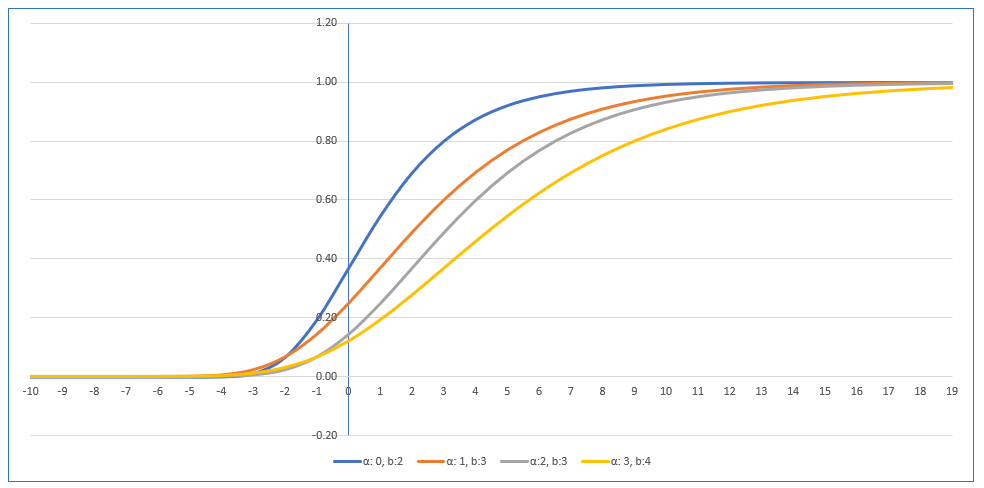# xlf Extreme Value distributions :: Gumbel

## 1. Gumbel distribution

Specifications (maximum):

• PDF Probability density (mass) function:
• $f(x) = \frac{1}{\beta} e^{-\frac{x- \alpha}{\beta}} e^{-e^{-\frac{x- \alpha}{\beta}}} = \frac{1}{\beta} exp \left[ - \left( \frac{x- \alpha} {\beta} + exp \left( \frac{x- \alpha} {\beta} \right) \right) \right]$
• CDF Cumulative density function (maximum): $F(x) = exp \left[- exp \left( \frac{x- \alpha} {\beta} \right) \right]$
• where $\alpha$ is the location parameter, and $\beta$ is the scale parameter, $\beta \gt 0$

### 1.1 Gumbel PDF

Probability density (mass) distribution (figure 1a)Fig 1a: Gumbel density mass function - $\alpha = 0$, $\beta = a$ shown in blue

A WS cell formula is provided in figure 1a

Cumulative density plot (figure 1b)Fig 1b: Gumbel density - WS formula =(1/R30C) * EXP(-( ((RC3 - R29C) / R30C) + EXP(-(RC3-R29C) / R30C) ))

### 1.1 Gumbel CDFFig 2a: Gumbel cumulative function- $\alpha = 0$, $\beta = a$ shown in blue

A WS cell formula is provided in figure 1b

## 2. VBA :: xlfGumbel function

There is no Excel built-in function for the Gumbel distribution. VBA code is provided in code 1 (xlfGumbel).

Code 1: xlfGumbel function
Function xlfGumbel(X As Double, Alpha As Double, Beta As Double, Cumulative As Boolean) As Variant
Dim tmp As Double
On Error GoTo ErrHandler

If Beta <= 0 Then GoTo ErrNum

If Cumulative = True Then
' cumulative distribution function
tmp = Exp(-Exp(-(X - Alpha) / Beta))
Else
' probability mass function
tmp = (1 / Beta) * Exp(-(((X - Alpha) / Beta) + Exp(-(X - Alpha) / Beta)))
End If

xlfGumbel = tmp
Exit Function
ErrNum:
xlfGumbel = VBA.CVErr(xlErrNum)
Exit Function
ErrHandler:
xlfGumbel = VBA.CVErr(xlErrValue)
End Function